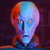# How to move the center (centroid) of a circle

370
2
02-26-2022 02:24 PMOccasional Contributor

Is there any way to move the center (centroid) of a circle/polygon to a new X/Y on the map, such as the current mouse location?

I have a solution that I found at https://csharp.hotexamples.com/examples/-/PolygonBuilder/-/php-polygonbuilder-class-examples.html under example #5 (CreateSearchPolygon), but unfortunately this causes too much overhead on the mouse move event that I am using.  There is a good amount of asynchronous code that is running, which causes major slow downs.  With that being said, there must be a simple solution that simply gets the new mouse point and moves the circle/polygon to that location.  It seems like an easy thing to do and should remove the need for any asynchronous code.  I just don't know what that solution would be.

Any assistance would be greatly appreciated.

Tags (4)
1 Solution

Accepted SolutionsOccasional Contributor

I have devised a solution for this problem with the assistance of the Esri ArcGIS Pro SDK team. I am utilizing the GeometryEngine.Instance.Move method on the polygon along with a delta X and delta Y coordinate.  The previous mouse point X/Y is subtracted from the current mouse point X/Y.  This creates the delta that is needed to move the centroid of the polygon to the correct location.  The following is the necessary code:

MapPoint screenMapPt = MapView.Active.ScreenToMap(new Point(e.X, e.Y));
MapPoint prevPt = MapView.Active.ScreenToMap(new Point(_prevX, _prevY));

ArcGIS.Core.Geometry.Geometry buffer = GeometryEngine.Instance.Move(_graphics[i].Polygon, (screenMapPt.X - prevPt.X), (screenMapPt.Y - prevPt.Y));
_graphics[i].Polygon = (Polygon)buffer;

The code is still a little bit laggy, since it all happens on the mouse move event, but it is faster than the previous option of creating a GeodesicBuffer.

Thanks again to the Esri ArcGIS Pro SDK team.

2 RepliesOccasional Contributor

I have a new solution that I found that builds a buffer polygon using GeometryEngine.Instance.GeodesicBuffer. It takes a point , a radius, and linear units and outputs a polygon.  The results are exactly what I need, but unfortunately I have to call it on every mouse move.  This still causes too much overhead, because the method is very slow and the polygon drags behind the mouse.  I should be able to create the geodesic buffer (polygon) once on an initial button click and then just move that buffer (polygon) at its centroid to a new location on the mouse move.  However, I still cannot do this.

The following is the current code that I am using:

System.Drawing.Point currentLoc = System.Windows.Forms.Control.MousePosition;
MapPoint mapPt = MapView.Active.ScreenToMap(new Point(currentLoc.X, currentLoc.Y));
ArcGIS.Core.Geometry.Geometry buffer = GeometryEngine.Instance.GeodesicBuffer(mapPt,      _graphics[i].Radius, GetLinearUnits(_graphics[i].Units));
if (_graphics[i].Graphic == null)
{
}
else
{
MapView.Active.UpdateOverlay(_graphics[i].Graphic, buffer, _graphics[i].Symbol);
}Occasional Contributor

I have devised a solution for this problem with the assistance of the Esri ArcGIS Pro SDK team. I am utilizing the GeometryEngine.Instance.Move method on the polygon along with a delta X and delta Y coordinate.  The previous mouse point X/Y is subtracted from the current mouse point X/Y.  This creates the delta that is needed to move the centroid of the polygon to the correct location.  The following is the necessary code:

MapPoint screenMapPt = MapView.Active.ScreenToMap(new Point(e.X, e.Y));
MapPoint prevPt = MapView.Active.ScreenToMap(new Point(_prevX, _prevY));

ArcGIS.Core.Geometry.Geometry buffer = GeometryEngine.Instance.Move(_graphics[i].Polygon, (screenMapPt.X - prevPt.X), (screenMapPt.Y - prevPt.Y));
_graphics[i].Polygon = (Polygon)buffer;

The code is still a little bit laggy, since it all happens on the mouse move event, but it is faster than the previous option of creating a GeodesicBuffer.

Thanks again to the Esri ArcGIS Pro SDK team.